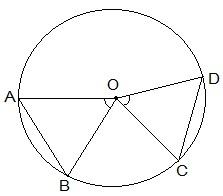# NCERT Solutions For Class 9 Math Chapter – 10 Exercise – 10.2

1. Recall that two circles are congruent if they have the same radii. Prove that equal chords of congruent circles subtend equal angles at their centres.

Solution:

To recall, a circle is a collection of points whose every point is equidistant from its centre. So, two circles can be congruent only when the distance of every point of both the circles are equal from the centre.For the second part of the question, it is given that AB = CD i.e. two equal chords.

Now, it is to be proven that angle AOB is equal to angle COD.

Proof:

Consider

the triangles ΔAOB and ΔCOD,

OA = OC and OB = OD (Since they are the radii of the circle)

AB = CD (As given in the question)

So, by SSS congruency, ΔAOB ΔCOD

∴ By CPCT we have,

AOB = COD. (Hence proved).

2. Prove that if chords of congruent circles subtend equal angles at their centres, then the chords are equal.

Solution:

Consider the following diagram-Here, it is given that AOB = COD i.e. they are equal angles.

Now, we will have to prove that the line segments AB and CD are equal i.e. AB = CD.

Proof:

In triangles AOB and COD,

AOB = COD (as given in the question)

OA = OC and OB = OD (these are the radii of the circle)

So, by SAS congruency, ΔAOB ΔCOD.

∴ By the rule of CPCT, we have

AB = CD. (Hence proved).